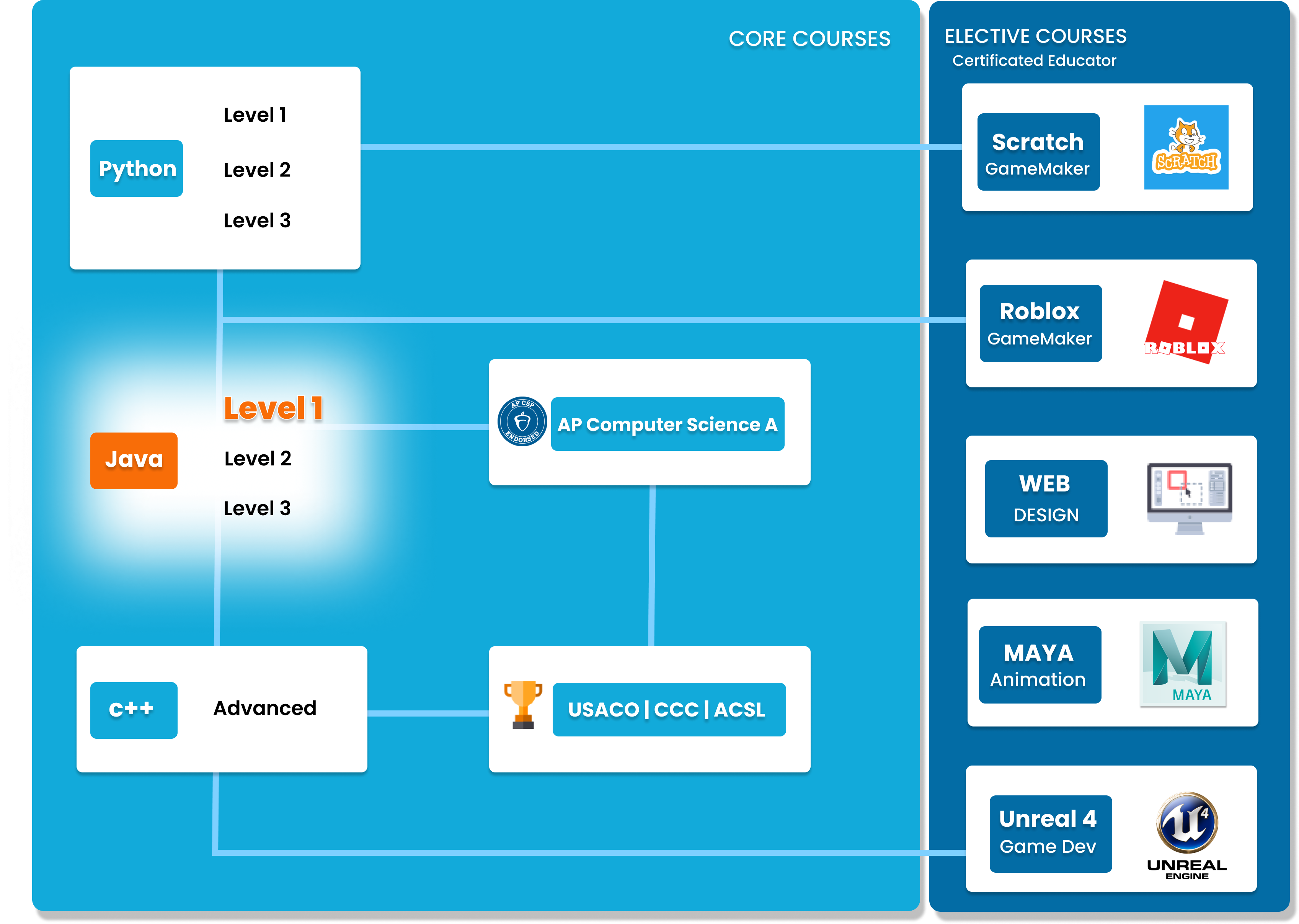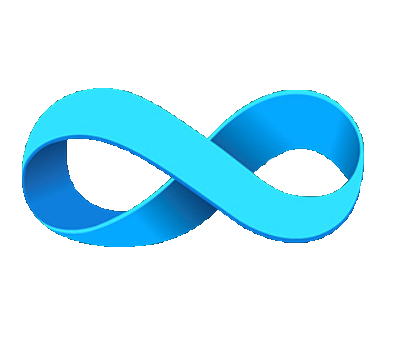# Java Level 1

In this beginner-friendly course, students will be introduced to the Java programming language. Students will learn the fundamentals of the language.##### Who is this course for
Beginner Students: This course is for students with little to no previous Java experience.
This course typically takes 3-6 months to complete. This depends on the student's experience and how fast they can master the concepts and the knowledge. Our instructors move at the pace of the student, it may take extra time for some students to reinforce what they have learned.
##### Curriculum
###### Chapter 1: Types and IdentifiersChapter 1 begins with the basics of variables: primitive types and identifiers. Additionally, students will learn about Boolean Algebra and how variables are stored in memory.
###### Chapter 2: OperatorsStudents will learn how to use arithmetic operators and its rules in Java, and learn about boolean expressions.
###### Chapter 3: Input/Output and Documentations.Chapter 3 focuses on user input and output: how to use the Scanner class, print formatting, and escape sequences. Additionally, students will learn about JavaDocs and commenting.
###### Chapter 4: Control StructuresIn chapter 4, students will learn about control structures such as conditionals and iterative loops.
###### Chapter 5: Errors and ExceptionsChapter 5 dives into errors and exception handling, featuring how to use the try-catch block and talks about the different types of errors and exceptions.
###### Chapter 6: Practice ExamsChapter 6 is a review of all the concepts learned in the previous chapters. Students will partake in mock exams in order to check their understanding, and ensure they are ready for the next level.

### You Might Also Be Interested In Our Elective Courses##### Introduction to Number Theory
AGES 10 - 15

This course is designed to build the basics of a complicated mathematical field. It is designed to be a complete introduction to the fundamental concepts of number theory for students in grades 7-10.

The class covers topics such as primes and composites; multiples, divisors, and divisibility; prime factorization and its uses; modular arithmetic and more.##### Introduction to Geometry
AGES 10 - 15

Students in grades 6-10 will build a solid understanding of more advanced geometry concepts in this course. It is designed to expand on the standard high school geometry curriculum and to challenge students with new concepts and problems.

Topics include congruent and similar triangles, circles, 3D geometry, and introductory trigonometry.##### Introduction to Counting and Probability
AGES 10 - 15

To build on the concepts from the Introduction level course, students will build on their Counting and Probability knowledge.

A thorough introduction for students in grades 7-10 to counting and probability topics such as permutations, combinations, Pascal's triangle, geometric probability, basic combinatorial identities, the Binomial Theorem, Pigeonhole Principle Conditional, Probability Graph Theory and more.